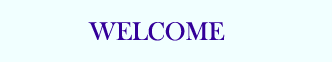TUTORIALS

## PHP Basic

PHP HOME
PHP Intro
PHP Install
PHP Syntax
PHP Variables
PHP String
PHP Operators
PHP If...Else
PHP Switch
PHP Arrays
PHP While Loops
PHP For Loops
PHP Functions
PHP Forms
PHP \$_GET
PHP \$_POST

PHP Date
PHP Include
PHP File
PHP Sessions
PHP E-mail
PHP Secure E-mail
PHP Error
PHP Exception
PHP Filter

## PHP Database

MySQL Introduction
MySQL Connect
MySQL Create
MySQL Insert
MySQL Select
MySQL Where
MySQL Order By
MySQL Update
MySQL Delete
PHP ODBC

XML Expat Parser
XML DOM
XML SimpleXML

AJAX Intro
AJAX PHP
AJAX Database
AJAX XML
AJAX Live Search
AJAX Poll

PHP Array
PHP Calendar
PHP Date
PHP Directory
PHP Error
PHP Filesystem
PHP Filter
PHP FTP
PHP HTTP
PHP Libxml
PHP Mail
PHP Math
PHP Misc
PHP MySQL
PHP SimpleXML
PHP String
PHP XML
PHP Zip

PHP Quiz
PHP Exam

# PHP Looping - While Loops

Loops execute a block of code a specified number of times, or while a specified condition is true.

## PHP Loops

Often when you write code, you want the same block of code to run over and over again in a row. Instead of adding several almost equal lines in a script we can use loops to perform a task like this.

In PHP, we have the following looping statements:

• while - loops through a block of code while a specified condition is true
• do...while - loops through a block of code once, and then repeats the loop as long as a specified condition is true
• for - loops through a block of code a specified number of times
• foreach - loops through a block of code for each element in an array

## The while Loop

The while loop executes a block of code while a condition is true.

### Syntax

 while (condition)   {   code to be executed;   }

### Example

The example below defines a loop that starts with i=1. The loop will continue to run as long as i is less than, or equal to 5. i will increase by 1 each time the loop runs:

 ";   \$i++;   } ?>

Output:

 The number is 1 The number is 2 The number is 3 The number is 4 The number is 5

## The do...while Statement

The do...while statement will always execute the block of code once, it will then check the condition, and repeat the loop while the condition is true.

### Syntax

 do   {   code to be executed;   } while (condition);

### Example

The example below defines a loop that starts with i=1. It will then increment i with 1, and write some output. Then the condition is checked, and the loop will continue to run as long as i is less than, or equal to 5:

 ";   } while (\$i<=5); ?>

Output:

 The number is 2 The number is 3 The number is 4 The number is 5 The number is 6

The for loop and the foreach loop will be explained in the next chapter.